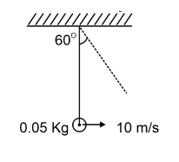NA
Question

# 4. Find the velocity of the ball when the string makes an angle of  60o with vertical. The string length is 2m and mass of the ball is 0.05 kg. Initially at the bottom most point the ball is given a velocity of 10 m/secModerate
Solution

## We have to apply the work energy theorem. Work done by gravity = change in kinetic energy.$\begin{array}{l}\mathrm{h}=2\left(1-\mathrm{Cos}{60}^{\circ }\right)=1\mathrm{m}\\ \frac{1}{2}\mathrm{m}\left({10}^{2}-{\mathrm{v}}^{2}\right)=\mathrm{mg}×1\\ ⇒{\mathrm{v}}^{2}=80⇒\mathrm{v}=4\sqrt{5}\mathrm{m}/\mathrm{sec}\end{array}$

Get Instant Solutions